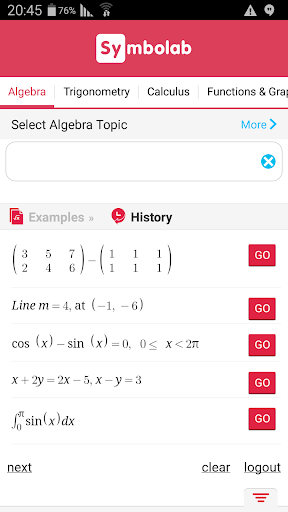Symbolab – Math solver [Subscribed]
Requirements: Android 3.0 and up
Overview: Your private math tutor, solves any math problem with steps! and all this without having Symbolap premium accountHistory! Every problem you solved is saved in history and you can get back to it anytime you want.

Symbolab calculator solves:
Algebra
* Equations
* Inequalities
* System of Equations
* Basic Operations (factor, join, cancel, simplify)
* Partial Fractions
* Polynomial Division
* Percents

Functions & Graphing
* Line equations (line, slope, parallel, perpendicular, midpoint, distance)
* Functions (domain, range, intercepts, asymptotes, extreme points, periodicity, inverse)
* Function arithmetics and compositions

Trigonometry:
* Trig Equations
* Trig identities proving
* Trig function evaluation
* Trig simplification

Calculus
* Limits
* Integrals (definite, indefinite, multiple)
* Derivatives
* Partial derivatives
* Series
* ODE
* Laplace Transform
* Inverse Laplace Transform

Matrices & Vectors
* Matrix Multiply, Power
* Matrix Trace
* Matrix Transpose
* Matrix Determinant
* Matrix Inverse
* Matrix Rank
* Matrix Reduce
* Vector Scalar Multiplication
* Vector Cross Product
* Vector Magnitude
* Vector Angle
* Vector Unit
* Vector Projection
* Vector Scalar Projection

(and more topics are added constantly)
Get free solution to any problem. Upgrade to get unlimited access to all subjects with steps.
For middle school through college students, teachers and parents.

WHAT’S NEW

Fixes connectivity issues on some devices

`https://play.google.com/store/apps/details?id=com.devsense.symbolab`